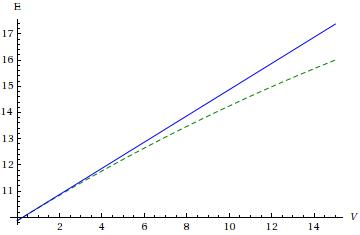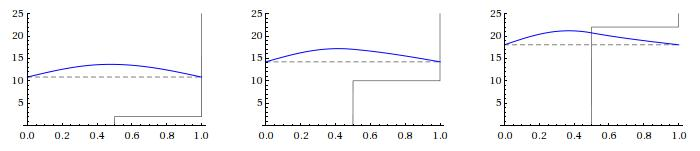[up] [top]

#### 3.1.1 Simple examples of perturbation theory

Probably the simplest example we can think of is an inﬁnite square well with a low step half way across, so that $V\left(x\right)=0$ for $0, ${V}_{0}$ for $a∕2 and inﬁnite elsewhere. We treat this as a perturbation on the ﬂat-bottomed well, so ${H}^{\left(1\right)}={V}_{0}$ for $a∕2 and zero elsewhere.

The ground-state unperturbed wavefunction is ${\psi }_{0}^{\left(0\right)}=\sqrt{\frac{2}{a}}sin\frac{\pi x}{a}$, with unperturbed energy ${E}_{0}^{\left(0\right)}={\pi }^{2}{\hslash }^{2}∕\left(2m{a}^{2}\right)$. A “low” step will mean ${V}_{0}\ll {E}_{0}^{\left(0\right)}$. Then we have

${E}_{0}^{\left(1\right)}=⟨{\psi }_{0}^{\left(0\right)}|{H}^{\left(1\right)}|{\psi }_{0}^{\left(0\right)}⟩=\frac{2}{a}{\int }_{a∕2}^{a}{V}_{0}{sin}^{2}\frac{\pi x}{a}\text{d}x=\frac{{V}_{0}}{2}$

This problem can be solved semi-analytically; in both regions the solutions are sinusoids, but with wavenumbers $k=\sqrt{2mE}∕\hslash$ and ${k}^{\prime }=\sqrt{2m\left(E-{V}_{0}\right)}∕\hslash$ respectively; satisfying the boundary conditions and matching the wavefunctions and derivatives at $x=a∕2$ gives the condition $kcot\left(ka∕2\right)={k}^{\prime }cot\left({k}^{\prime }a∕2\right)$ which can be solved numerically for $E$. (You ought to try this, it will be good practice for later sections of the course.) Below the exact solution (green, dotted) and ${E}_{0}^{\left(0\right)}+{E}_{0}^{\left(1\right)}$ (blue) are plotted; we can see that they start to diverge when ${V}_{0}=5$ (everything is in units of ${\hslash }^{2}∕\left(2m{a}^{2}\right)$).We can also plot the exact wavefunctions for diﬀerent step size, and see that for ${V}_{0}=10$ (the middle picture, well beyond the validity of ﬁrst-order perturbation theory) it is signiﬁcantly diﬀerent from a simple sinusoid.Another example is the harmonic oscillator, with a perturbing potential ${H}^{\left(1\right)}=\lambda {x}^{2}$. The states of the unperturbed oscillator are denoted $\left|\right{n}^{\left(0\right)}⟩$ with energies ${E}_{0}^{\left(0\right)}=\left(n+\frac{1}{2}\right)\hslash \omega$.

Recalling that in terms of creation and annihilation operators (see section A.4), $\stackrel{̂}{x}=\sqrt{\hslash ∕\left(2m\omega \right)}\left(â+{â}^{†}\right)$, with $\left[â,{â}^{†}\right]=1$, and so

${E}_{n}^{\left(1\right)}=⟨{n}^{\left(0\right)}|{H}^{\left(1\right)}|{n}^{\left(0\right)}⟩=\frac{\hslash \lambda }{2m\omega }⟨{n}^{\left(0\right)}|{\left({â}^{†}\right)}^{2}+{â}^{2}+2{â}^{†}â+1|{n}^{\left(0\right)}⟩=\frac{\lambda }{m{\omega }^{2}}\hslash \omega \left(n+\frac{1}{2}\right)$

The ﬁrst-order change in the wavefunction is also easy to compute, as $⟨{m}^{\left(0\right)}|{H}^{\left(1\right)}|{n}^{\left(0\right)}⟩=0$ unless $m=n±2$. Thus

$\begin{array}{rcll}\left|\right{n}^{\left(1\right)}⟩& =& \sum _{m\ne n}\frac{⟨{m}^{\left(0\right)}|{\stackrel{̂}{H}}^{\left(1\right)}|{n}^{\left(0\right)}⟩}{{E}_{n}^{\left(0\right)}-{E}_{m}^{\left(0\right)}}\left|\right{m}^{\left(0\right)}⟩& \text{}\\ & =& \frac{\hslash \lambda }{2m\omega }\left(\frac{\sqrt{\left(n+1\right)\left(n+2\right)}}{-2\hslash \omega }\left|\right{\left(n+2\right)}^{\left(0\right)}⟩+\frac{\sqrt{n\left(n-1\right)}}{2\hslash \omega }\left|\right{\left(n-2\right)}^{\left(0\right)}⟩\right)& \text{}\\ \text{and}\phantom{\rule{2em}{0ex}}{E}_{n}^{\left(2\right)}& =& ⟨{n}^{\left(0\right)}|{\stackrel{̂}{H}}^{\left(1\right)}|{n}^{\left(1\right)}⟩=\sum _{m\ne n}\frac{{\left|⟨{m}^{\left(0\right)}|{\stackrel{̂}{H}}^{\left(1\right)}|{n}^{\left(0\right)}⟩\right|}^{2}}{{E}_{n}^{\left(0\right)}-{E}_{m}^{\left(0\right)}}& \text{}\\ & =& {\left(\frac{\hslash \lambda }{2m\omega }\right)}^{2}\left(\frac{\left(n+1\right)\left(n+2\right)}{-2\hslash \omega }+\frac{n\left(n-1\right)}{2\hslash \omega }\right)=-\frac{1}{2}{\left(\frac{\lambda }{m{\omega }^{2}}\right)}^{2}\hslash \omega \left(n+\frac{1}{2}\right)& \text{}\end{array}$

We can see a pattern emerging, and of course this is actually a soluble problem, as all that the perturbation has done is change the frequency. Deﬁning ${\omega }^{\prime }=\omega \sqrt{1+2\lambda ∕\left(m{\omega }^{2}\right)}$, we see that the exact solution is

${E}_{n}=\left(n+\frac{1}{2}\right)\hslash {\omega }^{\prime }=\left(n+\frac{1}{2}\right)\hslash \omega \left(1+\frac{\lambda }{m{\omega }^{2}}-\frac{1}{2}{\left(\frac{\lambda }{m{\omega }^{2}}\right)}^{2}+\dots \right)$

in agreement with the perturbative calculation.

[up] [top]